# Arrays

Declaration, Allocation, and Initialization

Before it can be used, a variable must be both declared (to specify its type) and initialized (to give it an initial value). For a variable of an array type, there are three steps we must perform: declaration, allocation, and initialization. First, we must declare the variable. For example:

```int[] nums;
```

The second step is to allocate space for the array. We have to tell Java how many elements the array will have so that it can set aside the appropriate amount of memory. The syntax for allocation uses the keyword new:

```nums = new int;
```

The array now exists, but the elements themselves have not yet been initialized. They have default valuesin this case, they are all 0. We often use a for loop to initialize the elements:

```for (int i = 0; i < nums.length; i++) {
nums[i] = i * 2;
}
```

The effects of these steps are summarized in Figure 2-6.

Figure 2-6. Declaration, allocation, and initialization of an array variable. In the first diagram, the line ending in a dot indicates a null reference.

(This item is displayed on page 45 in the print version)

Code

UML instance diagram

```// Declaration
int[] nums;
``````// Allocation
nums = new int;
```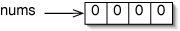```// Initialization of elements
for (int i = 0; i < nums.length; i++) {
nums[i] = i * 2;
}
```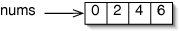We can perform all three of these steps in a single statement by explicitly supplying the values of the array elements. This is reasonable only for relatively short arrays:

```int[] nums = new int[] {0, 2, 4, 6};
```

The ability of a variable of an array type to hold an array of any size is another example of polymorphism.

Multidimensional Arrays

We can declare an array of anything. An array of Die objects would be declared like this:

```Die[] dice;
```

An array of ints would be declared like this:

```int[] nums;
```

We can even declare an array of arrays of ints, like this:

```int[][] rows;
```

An array of arrays is called a multidimensional array. Specifically, rows is a two-dimensional array, analogous to a Chess board. If we allocate rows with the statement

```rows = new int;
```

then we get the data structure shown in Figure 2-7.

Figure 2-7. Instance diagram showing a two-dimensional array. The shaded element is rows.The array rows is said to have dimensionality 2, because we have to specify two indices to get at a particular element. The dimensions of the array are 3 (the number of rows) and 4 (the number of columns).

While it would be difficult to draw, we could declare and allocate an array of dimensionality 4:

```int[][][][] tesseract = new int;
```

This array has dimensions 2, 5, 4, and 3. It is rare to see dimensionalities greater than 3, because such arrays quickly become impractically large. Even tesseract has 120 elements!

This array-of-arrays representation allows for several interesting tricks. If we supply only one index for rows, we get a reference to a single row of the array. For example, if we say

```int[] middleRow = rows;
```

we get the situation in Figure 2-8.

Figure 2-8. A reference to a single row of a two-dimensional array.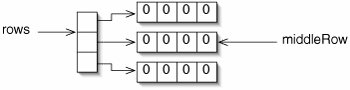We can also allocate an array one part at a time. For example,

```int[][] rows = new int[];
```

allocates the spine of the array, but not any of the rows. Since the elements of this array are references, they get the default value null. This is shown in Figure 2-9.

Figure 2-9. A two-dimensional array with only the spine allocated.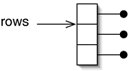Now we can allocate the first row with

```rows = new int;
```

giving the situation shown in Figure 2-10.

Figure 2-10. A two-dimensional array with the first row allocated.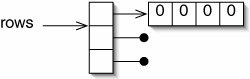There is no reason the other rows have to have the same length. If we now allocate

```rows = new int;
rows = new int;
```

we get a ragged array, as shown in Figure 2-11.

Figure 2-11. In a ragged array, different rows have different lengths.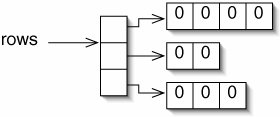Example: Domineering

To illustrate the use of arrays, we now write a program to let two people play the game of Domineering (Figure 2-12).

Figure 2-12. The game of Domineering, also known as Crosscram, was invented by Göran Andersson.

Domineering

Players: 2, one playing horizontally and one vertically.

Object: To be the last player with a legal move.

Board: The board is an 8 x 8 square grid, as in Chess or Checkers. It is initially empty.

Play: On a turn, a player places a domino on the board to occupy two adjacent squares. One player places his dominoes horizontally (east-west), the other vertically (north-south). The dots on the dominoes are ignored, but a domino cannot overlap any previously played dominoes.

What classes will we need? An initial sketch (Figure 2-13) suggests that we'll need a Domineering object, one Board object, and a number of Domino objects.

Figure 2-13. This UML class diagram says that a Domineering object is associated with one Board object and 0 to many Domino objects. The asterisk denotes "many." Our actual program will not have this structure.

(This item is displayed on page 48 in the print version)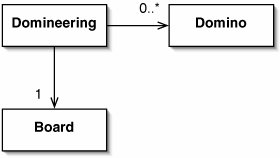Further thought reveals that this is overkill. While Domineering involves dominoes, they don't have any interesting state. We don't even care what numbers are on them. All they do is take up space on the board. As long as we keep track of which board squares are occupied, we don't really need a Domino class for this game.

In fact, the board is such a simple thing that we can represent it with a two-dimensional array of booleans. An individual element is true if that square is occupied. We can implement Domineering with a single class (Figure 2-14).

Figure 2-14. The Domineering game can be implemented with a single class. As always, static fields and methods are underlined. It is not yet obvious what all of the fields and methods are for, but we show them here for completeness.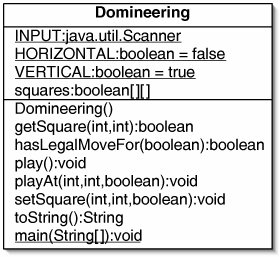The field squares, the constructor, and the main() method are shown in Figure 2-15.

After allocating squares, we could have initialized the elements with the code

```for (int row = 0; row < 8; row++) {
for (int column = 0; column < 8; column++) {
squares[row][column] = false;
}
}
```

but, since false is the default value for booleans, we don't have to do this.

Figure 2-15. A field, constructor, and main() method for Domineering.

 ``` 1 /** The game of Domineering. */ 2 public class Domineering { 3 4 /** Array of board squares, true if occupied. */ 5 private boolean[][] squares; 6 7 /** The board is initially empty. */ 8 public Domineering () { 9 squares = new boolean; 10 // Java initializes all array elements to false 11 } 12 13 /** Create and play the game. */ 14 public static void main(String[] args) { 15 System.out.println("Welcome to Domineering."); 16 Domineering game = new Domineering(); 17 game.play(); 18 } 19 20 } ```

The toString() method (Figure 2-16) uses nested for loops.

Figure 2-16. The toString() method uses nested for loops.

 ``` 1 public String toString() { 2 String result = " 0 1 2 3 4 5 6 7"; 3 for (int row = 0; row < 8; row++) { 4 result += " " + row; 5 for (int column = 0; column < 8; column++) { 6 if (squares[row][column]) { 7 result += " #"; 8 } else { 9 result += " ."; 10 } 11 } 12 } 13 return result; 14 } ```

Figure 2-17 shows a String the method might return for a board where one horizontal and one vertical domino have been placed.

As in BeetleGame, the play() method contains a loop which repeats until the game is over. This method must keep track of who the current player is. Rather than numbering the players and trying to remember which one plays horizontally and which one vertically, we define two

Figure 2-17. Typical output of the toString() method.

 ```1 0 1 2 3 4 5 6 7 2 0 . # . . . . . . 3 1 . # . . . . . . 4 2 . . . . . . . . 5 3 . . . . . . . . 6 4 . . . . . . . . 7 5 . . . . . # # . 8 6 . . . . . . . . 9 7 . . . . . . . . ```

constants HORIZONTAL and VERTICAL. This makes the code easier to read. Since there are only two choices, we can use the boolean values false and true for these constants.

An added advantage of this representation is that we can switch players with the simple statement:

```player = !player;
```

The play() method and these constants are shown in Figure 2-18.

Figure 2-18. The play() method and associated constants. On line 15, we want to print a blank line above the diagram of the board. We do this by adding the newline String " " to this (which is implicitly this.toString()) and passing the result to System.out.println().

 ``` 1 /** For reading from the console. */ 2 public static final java.util.Scanner INPUT 3 = new java.util.Scanner(System.in); 4 5 /** The player who plays their dominoes horizontally. */ 6 public static final boolean HORIZONTAL = false; 7 8 /** The player who plays their dominoes vertically. */ 9 public static final boolean VERTICAL = true; 10 11 /** Play until someone wins. */ 12 public void play () { 13 boolean player = HORIZONTAL; 14 while (true) { 15 System.out.println(" " + this); 16 if (player == HORIZONTAL) { 17 System.out.println("Horizontal to play"); 18 } else { 19 System.out.println("Vertical to play"); 20 } 21 if (!(hasLegalMoveFor(player))) { 22 System.out.println("No legal moves -- you lose!"); 23 return; 24 } ``` 25 System.out.print("Row: "); 26 int row = INPUT.nextInt(); 27 System.out.print("Column: "); 28 int column = INPUT.nextInt(); 29 playAt(row, column, player); 30 player = !player; 31 } 32 }

The play() method invokes two other methods, hasLegalMoveFor() and playAt(). The first determines if there is any legal move left for the current playerif not, the game is over. The second actually updates the array squares.

We present playAt() first, as it is simpler. This method (Figure 2-19) sets two elements of squares to true.

Figure 2-19. The playAt() method actually modifies the elements of squares. Two elements are modified: one in line 5 and one in either line 7 or line 9.

 ``` 1 /** 2 * Play a domino with its upper left corner at row, column. 3 */ 4 public void playAt(int row, int column, boolean player) { 5 squares[row][column] = true; 6 if (player == HORIZONTAL) { 7 squares[row][column + 1] = true; 8 } else { 9 squares[row + 1][column] = true; 10 } 11 } ```

The hasLegalMoveFor() method is more complicated, because it has to act slightly differently depending on the current player. If it is looking for horizontal moves, it has to check rows 0 through 7 and columns 0 through 6, making sure that both

```squares[row][column]
```

and

```squares[row][column + 1]
```

are unoccupied. On the other hand, when looking for vertical moves, it has to check rows 0 through 6 and columns 0 through 7, making sure that both

```squares[row][column]
```

and

```squares[row + 1][column]
```

are unoccupied. Rather than write loops for each of these very similar cases, we write the loops once, using variables rowOffset and columnOffset to control which version we use. Thus, the second square we check is:

```squares[row + rowOffset][column + columnOffset]
```

If player is HORIZONTAL, rowOffset is 0 and columnOffset is 1. If player is VERTICAL, rowOffset is 1 and columnOffset is 0.

These variables are also used in the termination tests in the for loops. The hasLegalMoveFor() method is shown in Figure 2-20.

Figure 2-20. The exact behavior of the nested for loop in lines 1219 of hasLegalMoveFor() is controlled by the variables rowOffset and columnOffset.

 ``` 1 /** 2 * Return true if there is a legal move for the specified player. 3 */ 4 public boolean hasLegalMoveFor(boolean player) { 5 int rowOffset = 0; 6 int columnOffset = 0; 7 if (player == HORIZONTAL) { 8 columnOffset = 1; 9 } else { 10 rowOffset = 1; 11 } 12 for (int row = 0; row < (8 - rowOffset); row++) { 13 for (int column = 0; column < (8 - columnOffset); column++) { 14 if (!(squares[row][column] 15 // squares[row + rowOffset][column + columnOffset])) { 16 return true; 17 } 18 } 19 } 20 return false; 21 } ```

We conclude the example with testing. The first few turns of a game of Domineering are shown in Figure 2-21.

Figure 2-21. Beginning a game of Domineering. Text typed by the user is in grey.

 ``` 1 Welcome to Domineering. 2 3 0 1 2 3 4 5 6 7 4 0 . . . . . . . . 5 1 . . . . . . . . 6 2 . . . . . . . . 7 3 . . . . . . . . 8 4 . . . . . . . . 9 5 . . . . . . . . 10 6 . . . . . . . . 11 7 . . . . . . . . 12 Horizontal to play 13 Row: 1 14 Column: 0 15 16 0 1 2 3 4 5 6 7 17 0 . . . . . . . . 18 1 # # . . . . . . 19 2 . . . . . . . . 20 3 . . . . . . . . 21 4 . . . . . . . . 22 5 . . . . . . . . 23 6 . . . . . . . . 24 7 . . . . . . . . 25 Vertical to play 26 Row: 5 27 Column: 6 28 29 0 1 2 3 4 5 6 7 30 0 . . . . . . . . 31 1 # # . . . . . . 32 2 . . . . . . . . 33 3 . . . . . . . . 34 4 . . . . . . . . 35 5 . . . . . . # . 36 6 . . . . . . # . 37 7 . . . . . . . . 38 Horizontal to play ```

Exercises

2.10

Draw a UML instance diagram of the situation after evaluating the code below.

```int[] arr = new int;
int[] avast = new int;
int[] shiverMeTimbers = arr;
int[] yoHoHo;
arr = 5;
avast = 8;
```

2.11

Draw a UML instance diagram of the data structure produced by the code below.

```int[][] triangle = new int[][] {{1, 2, 3}, {4, 5}, {6}};
```

2.12

Suppose we need to store a table of distances between cities, as found in a road atlas. One obvious approach would be to use a square array distances, where distances[i][j] is the distance between city i and city j. Explain how to use a ragged array to cut the amount of memory needed for this data structure roughly in half.

2.13

If the array arr has dimensions 3 and 7, what is arr.length?

2.14

Is the statement below legal? Explain.

```Object[] ref = new int;
```

2.15

Arrays are not objects, so we can't invoke methods on them. This can make it awkward to, for example, test two arrays for equality. The built-in java.util.Arrays class provides several static methods that work on arrays. Look this class up in the API. Explain the difference between the equals() and deepEquals() methods and between the toString() and deepToString() methods. (If you use it in code, you must refer to the Arrays class as java.util.Arrays, for reasons explained in Chapter 3.)

2.16

Draw a UML instance diagram of the data structures that exist just before exiting the main() method in Figure 2-22.

Figure 2-22. Code for Exercise 2.16.

 ```1 public static void main(String[] args) { 2 int[][] numbers = new int[]; 3 int[] row = new int[] {0, 1, 2, 3}; 4 for (int i = 0; i < numbers.length; i++) { 5 numbers[i] = row; 6 } 7 } ```
2.17

Suppose we provided an accessor getSquares() for the Domineering class. A method in another class, given an instance game, might do this:

```game.getSquares() = true;
```

Discuss whether this violates encapsulation.

2.18

As written, the Domineering program does not verify that a player has chosen a valid location. A player may place a domino so that it overlaps an existing one. Also, if the player places a domino so that part of it is off the board, the program will crash. Modify the program to fix both these problems. (If the player enters invalid coordinates, give her a chance to enter valid ones.)

2.19

Domineering is normally played on an 8 x 8 board, but there is no reason it couldn't be played on a 4 x 4 or 10 x 10 board. Modify the program to allow the user to specify the board size. You will need to eliminate all mention of the magic number 8 from the program. (Hint: Instead of storing the board size in a separate field, you can simply use squares.length.)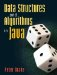Data Structures and Algorithms in Java
ISBN: 0131469142
EAN: 2147483647
Year: 2004
Pages: 216
Authors: Peter Drake